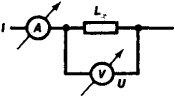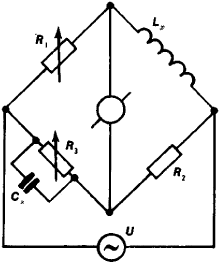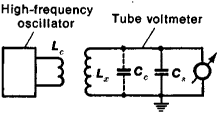# Inductance Meters

The following article is from The Great Soviet Encyclopedia (1979). It might be outdated or ideologically biased.

## Inductance Meters

instruments for measuring the inductance of circuits with lumped parameters, the windings of transformers and chokes, and inductance coils. Their principle of operation depends on the method of measurement.Figure 1. Diagram of the measurement of inductance by the “voltmeter-ammeter” method:(A) ammeter, (V) voltmeter, (Lx) inductance, (l) current, (U) voltage

The “voltmeter-ammeter” method (Figure 1) is used for measuring relatively large inductances (from 0.1 to 1,000 henrys [H]) when the resistance of the windings is significantly lower than the inductance. In this case Lx = U/(π f · I), where U is the voltage, I is the current in the circuit whose inductance is being measured, and f is the frequency of the alternating current, usually 50 hertz (Hz). Such inductance meters have an error of 2–3 percent.

An AC measuring bridge (for frequencies of 100, 400, and 1,000 Hz) with standard capacitance or, much less frequently,Figure 2. Diagram of a bridge for measuring inductance: (U) current source; (G) galvanometer; (R3), (R2), and (R3) ohmic resistances; (Cx,) standard capacitance; (Lx) inductance being measured

standard inductance (Figure 2) is the main component of inductance meters. When the bridge is balanced,

rx = (R1· R2)/R3Lx = Cs· R1· R2

where rx is the ohmic resistance of the wire of the inductance coil winding. Bridge measurement methods have an error of 1–3 percent; the range of measurement is 0.1 to 1,000 H.

Resonance methods are based on the resonant properties of an oscillatory circuit composed of the inductance being measured (Lx) and a standard capacitance Cs (Figure 3). By varying C„ the circuit can be tuned to resonance with the generator (at frequencies of 10 kHz to 1.5 MHz); the inductance is then calculated using the formula Lx = (2.53 ×l0)(f20 · c), where Lx is the inductance in mH, f0 is the resonance frequency in kHz, and C = Cs + Cc is the total capacitance of the circuit in picofarads. Resonant inductance meters have an error of 2–5 percent; the range of measurement is 0.05 μ.H to 100 mH.Figure 3. Diagram of resonance method of inductance measurement: (Lc) coupling loop, (Lx) inductance being measured, (Cc) self-capacitance of coil, (Cs) standard capacitance

### REFERENCE

Shkurin, G. P. Spravochnik po elektroizmeritel’nym i radioizmeritel’nympriboram, 3rd ed., vol. 2. Moscow, 1960.